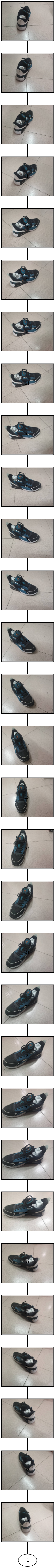# 计算机视觉中的特征点检测与匹配

**角点检测(Corner Detection)**是计算机视觉系统中用来获得图像特征的一种方法，广泛应用于运动检测、图像匹配、视频跟踪、三维建模和目标识别等领域中。也称为特征点检测。

## 1.Harris角点检测算法``````# Harris角点提取
def extraHarrisfromImg(img, threshold):
if img.ndim == 3:
img = cv2.cvtColor(img, cv2.COLOR_RGB2GRAY)
img = np.float32(img)
# opencv函数计算响应值
dst = cv2.cornerHarris(img, 2, 3, 0.04)
# 实际阈值
threshold = threshold*dst.max()
# 大于阈值的设为角点
cor_axis = np.array(np.where(dst > threshold)).T[:,[1,0]]
# 返回角点坐标
return cor_axis
``````

``````# 可视化角点
def drawCor(img, cors):
red = (0,0,255)
gre = (0,255,0)
yel = (0,255,255)

if cors.shape == 4:
... ...
else:
for cor in cors:
#绘制角点
x0, y0 = int(round(cor)), int(round(cor))
cv2.circle(img, (x0, y0), 5, yel, 1, lineType=cv2.LINE_AA)
``````

``````    data_root, result_root = './datasets/', './cor_info/'
img1,_,_ = utils.auto_reshape(img1, 820)
harris_cors = extraHarrisfromImg(img1, 0.1)
print(harris_cors.shape)
drawCor(img1, harris_cors)
``````

(5596, 2)

(3365, 2)

(586, 2)

Harris角点提取结果以及响应图可视化：## 2.SIFT角点检测算法

SIFT即尺度不变特征转换(Scale-invariant feature transform)

### 使用VLFeat开源库进行SIFT特征提取``````sift [img_file] --output=[output_file] --edge-thresh 10 --peak-thresh 5
``````peak参数越大，算法越能够过滤掉在DOG尺度空间中图像特征下响应程度较小的极值点edge参数越小，越能够过滤掉DOG尺度空间中曲率越小的极值点(越容易是边缘的特征点)

``````def extraSIFTfromImg(src_name, src):
# 转化为灰度图
im = cv2.cvtColor(src, cv2.COLOR_RGB2GRAY)
# 灰度图另存为
img_name = 'tmp.pgm'
cv2.imwrite(img_name, im)
dst_name = src_name + '.sift'
cmd_param = ' --edge-thresh 10 --peak-thresh 5'
command = 'sift ' + img_name + ' --output=' + dst_name + cmd_param
# 执行命令行命令
os.system(command)
``````

``````    img = cv2.imread('home.jpg')[:,:, [2,1,0]]
# 图像reshape
img, h, w = utils.auto_reshape(img, 1080)
extraSIFTfromImg('home', img)
``````

``````# 读取文本文件中的SIFT特征并转化为numpy数组
def txt2SIFT(name, root='./c/'):
x, y, scale, direction, desc = [], [], [], [], []
for cor in cors:
cor = cor.split(' ')[:-1] # 去除掉换行符
x.append(float(cor))          # 坐标x
y.append(float(cor))          # 坐标y
scale.append(float(cor))      # 尺度
direction.append(float(cor))  # 方向
desc.append([int(i) for i in cor[4:]]) # 描述子
cors_info = np.array([x,y,scale,direction]).T
desc = np.array(desc)
return cors_info, desc
``````SIFT特征可视化：

``````# 可视化角点
def drawCor(img, cors):
red = (0,0,255)
gre = (0,255,0)
yel = (0,255,255)

if cors.shape == 4:
for cor in cors:
θ = cor             # 方向
r = int(round(cor)) # 尺度
x0, y0 = int(round(cor)), int(round(cor)) # 坐标
x1, y1 = int(round(x0 + r*np.cos(θ))),  int(round(y0 + r*np.sin(θ)))
# 绘制角点
cv2.circle(img, (x0, y0), r, gre, 1, lineType=cv2.LINE_AA)
cv2.line(img, (x0,y0), (x1, y1), red, 1, lineType=cv2.LINE_AA)
else:
... ...
cv2.imwrite('corner.png', img)
``````

``````    data_root, result_root = './datasets/', './cor_info/'
# 批量提取sift特征
# extractBatchSIFTs(data_root, result_root)
img1,_,_ = utils.auto_reshape(img1, 1080)
sift_cors, desc = extraSIFTfromImg(img1, img_name)
print(sift_cors.shape)
drawCor(img1, sift_cors)
``````

(1603, 4)### SIFT特征与Harris特征对比

``````img1 = cv2.imread('./corner.png')[:,:,[2,1,0]]
imgs = {'SIFT edge=5 peak=10':img1,'Harris T=0.5':img2,}
utils.view_contrast(imgs)
``````### 图像间的SIFT特征点匹配

``````# 行数据标准化
def norm(x):
return x / np.linalg.norm(x,axis=1).reshape(-1,1)
``````

``````# 描述子单向匹配
def singleMatch(norm_desc1, norm_desc2):
match_seq = []  # 匹配序列
# 计算余弦距离
sim_matrix = norm_desc2 @ norm_desc1.T
sim_matrix = sim_matrix.T # 相似矩阵
# 可视化相似矩阵
# plt.imshow(sim_matrix)
# plt.show()
# 从大到小顺序排列
sim_idx = np.argsort(sim_matrix, 1)[:,::-1]
for i in range(sim_idx.shape):
top1_idx, top2_idx = sim_idx[i,0], sim_idx[i,1]
top1_val = sim_matrix[i,top1_idx]
top2_val = sim_matrix[i,top2_idx]
# 最近邻角度/第二近邻角度<阈值 ? 是匹配点 : 舍弃
if np.arccos(top1_val) < 0.6 * np.arccos(top2_val):
match_seq.append([i, top1_idx])
else:
match_seq.append([i, -1])

return np.array(match_seq)
``````

``````        # 最近邻角度/第二近邻角度<阈值 ? 是匹配点 : 舍弃
if np.arccos(top1_val) < 0.6 * np.arccos(top2_val):
``````

``````# 描述子双向匹配
def doubleMatch(desc1, desc2):
# 标准归一化
norm_desc1 = norm(desc1)
norm_desc2 = norm(desc2)
# 双向匹配
matches_12 = singleMatch(norm_desc1, norm_desc2)
matches_21 = singleMatch(norm_desc2, norm_desc1)
matcher = []
for i in range(matches_12.shape):
if(matches_12[i,1] != -1): # 排除掉无匹配的点
# 若双向匹配是对称的，才是合格的匹配点
if matches_21[matches_12[i,1], 1] == matches_12[i,0]:
matcher.append([matches_12[i,0], matches_12[i,1]])
return np.array(matcher)
``````SIFT特征匹配效果：## 3.实战 校园建筑物匹配

``````# 批量提取图像SIFT特征
def extractBatchSIFTs(data_root, result_root):
for i in range(len(img_path)):
img, _, _ = utils.auto_reshape(img, 1080)
extraSIFT2txt(img, img_name[i], result_root)
``````

``````# 匹配与当前图像最相似的帧(用于拍摄时序还原)
def matchImgViz(data_root, result_root, downsample=False):
small_data_root = 'D:/YHT/学习/大三下/computer_vision/exp2/datasets_small/'
if(downsample):
for i in range(len(img_path)):
img,_,_ = utils.auto_reshape(img, 200)
cv2.imwrite('./datasets_small/' + img_name[i]+'.png', img)
print(small_data_root + img_name[i]+'.jpg')

g = pydot.Dot(graph_type='graph')
for i in range(match_matrix.shape-1):
for j in range(i+1,match_matrix.shape):
if(match_matrix[i,j]>1):
print(small_data_root + img_name[i]+'.png')
g.write_png('graph.jpg')
``````### 数据采集### 匹配结果可视化## 4.实战 视频流时序还原

``````# 批量进行图像SIFT特征匹配
def matchBatchImgs(data_root, result_root, extraSIFT=False):
if extraSIFT:
extractBatchSIFTs('./datasets/', './cor_info/')
img_nums = len(img_name)
# 获取该批图像的所有描述子:
desc_list = []
for i in range(img_nums):
_, desc = txt2SIFT(img_name[i], result_root)
desc_list.append(desc)
# 两两进行匹配：
match_matrix = np.zeros((img_nums, img_nums))
for i in tqdm(range(img_nums-1), desc='Processing'+str(i)):
j=i+1
for j in tqdm(range(i+1, img_nums), desc='Processing'+str(j)):
matches = doubleMatch(desc_list[i], desc_list[j])
match_matrix[i,j] = matches.shape
# 可视化匹配矩阵
origin_matrix = match_matrix
plt.imshow(match_matrix)
plt.show()
# 下面这段代码删除无匹配点的矩阵行
zero_rows = []
for i in range(img_nums):
if(sum(match_matrix[i,:]) == 0):
zero_rows.append(i)
match_matrix = np.delete(match_matrix, zero_rows, axis=0)
# 匹配索引矩阵:
match_idx_matrix = np.argsort(match_matrix, 1)[:,::-1]
match_sort_matrix = np.sort(match_matrix, 1)[:,::-1]
# 将那些0匹配的图像对设为-1，不再考虑
match_idx_matrix[match_sort_matrix==0] = -1
# 输出匹配图像的名称(无向图)
match_img_name = {}
for i in range(match_idx_matrix.shape):
names = []
for idx in match_idx_matrix[i,:]:
if idx == -1:break
names.append(img_name[idx])
match_img_name[img_name[i]] = names
print(match_img_name)
return origin_matrix# match_matrix   # 无向图邻接矩阵
``````

### 数据采集### 匹配结果可视化(0)